# Coordinate Plane Worksheets Spongebob

📆 7 Aug 2022
🔖 Other Category
📂 Gallery Type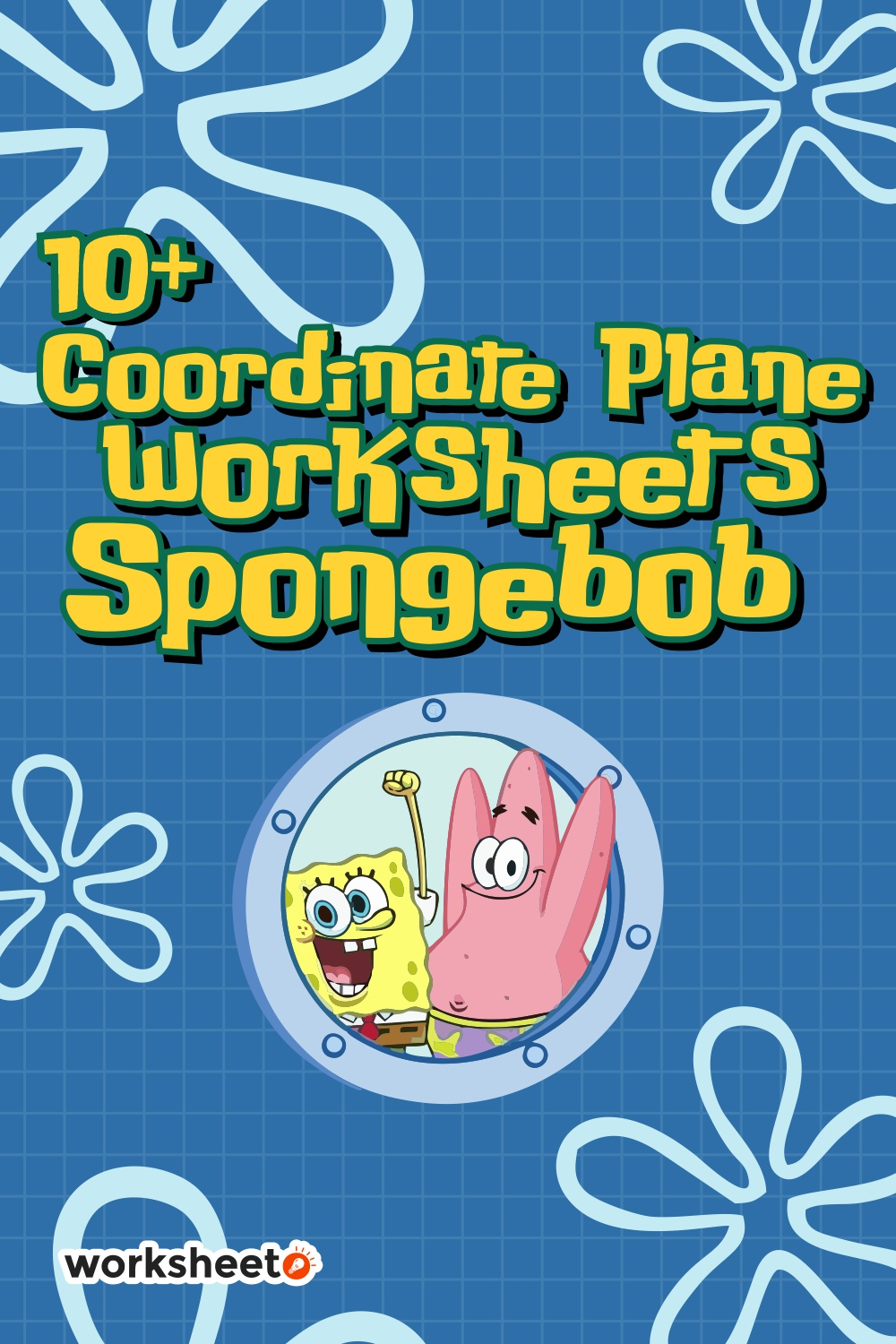17 Images of Coordinate Plane Worksheets Spongebob

See the complete Coordinate Plane Worksheets Spongebob here.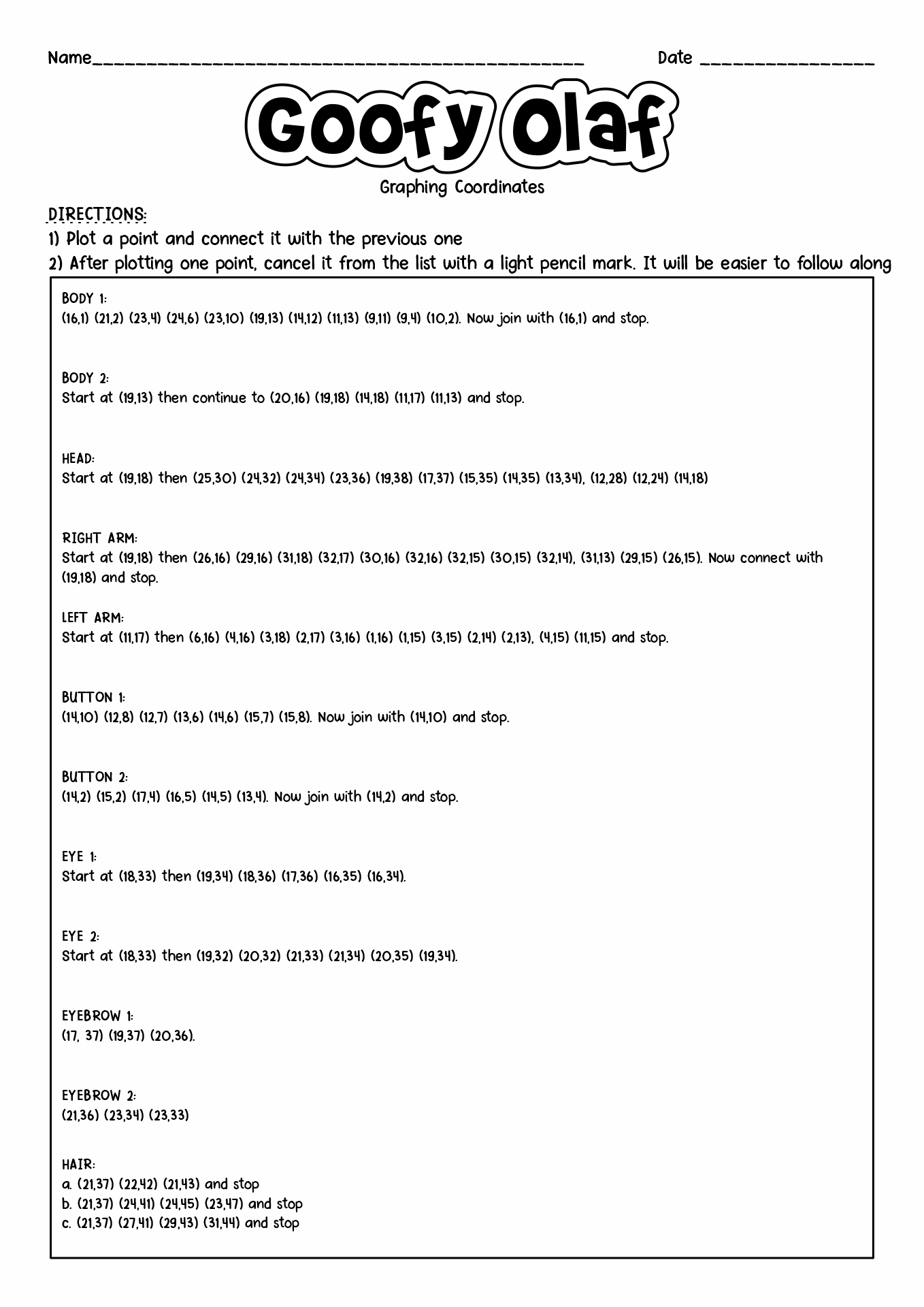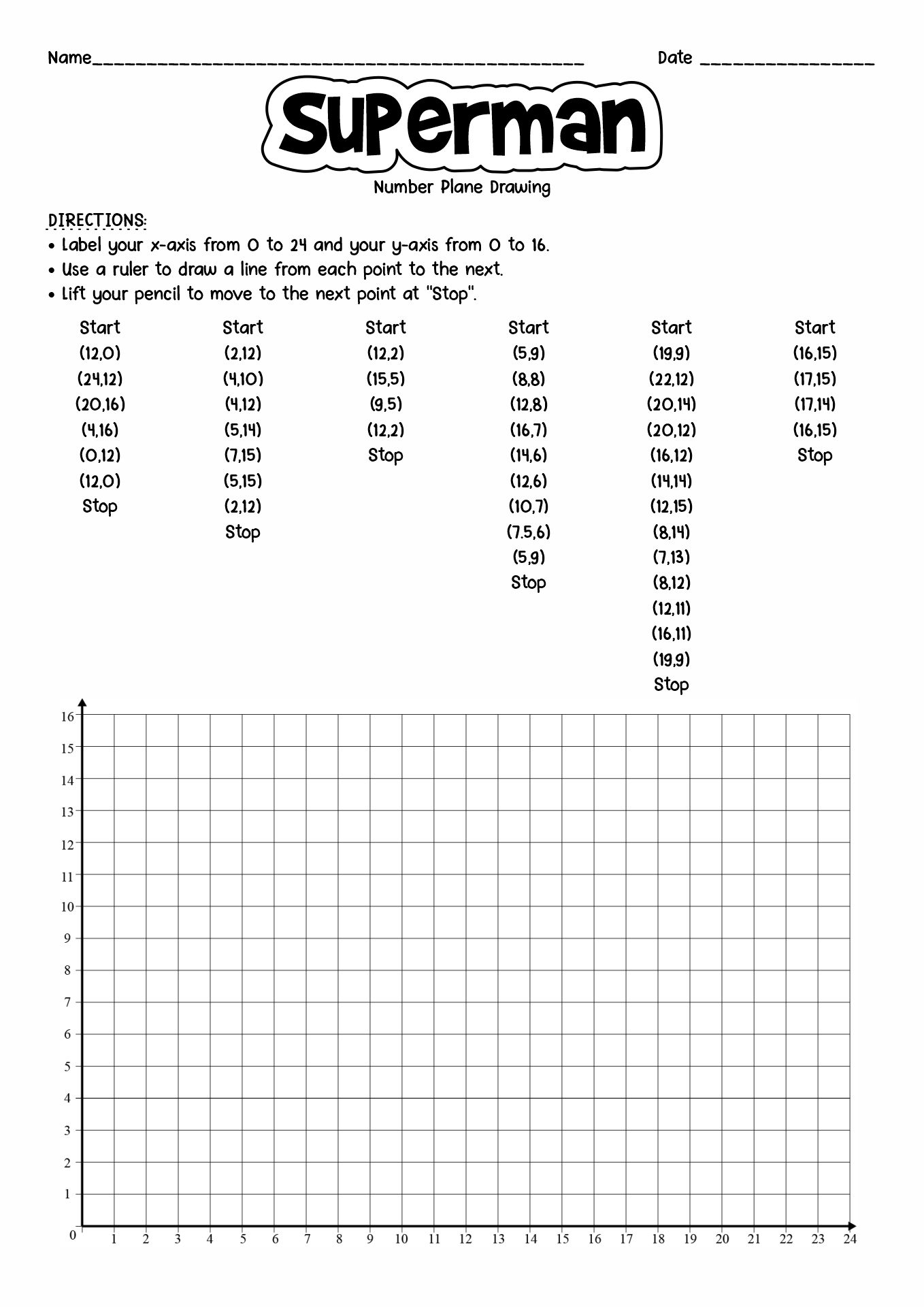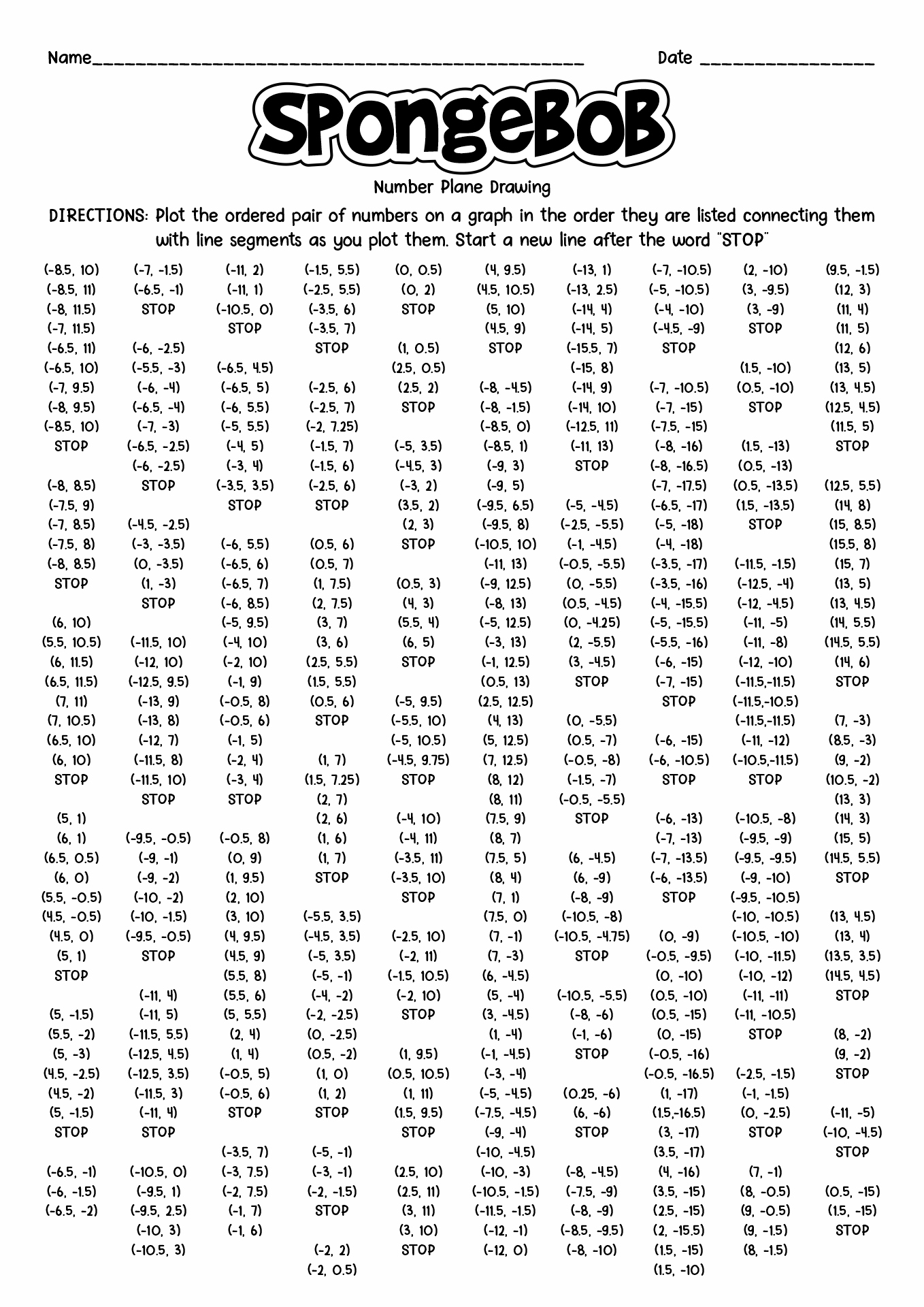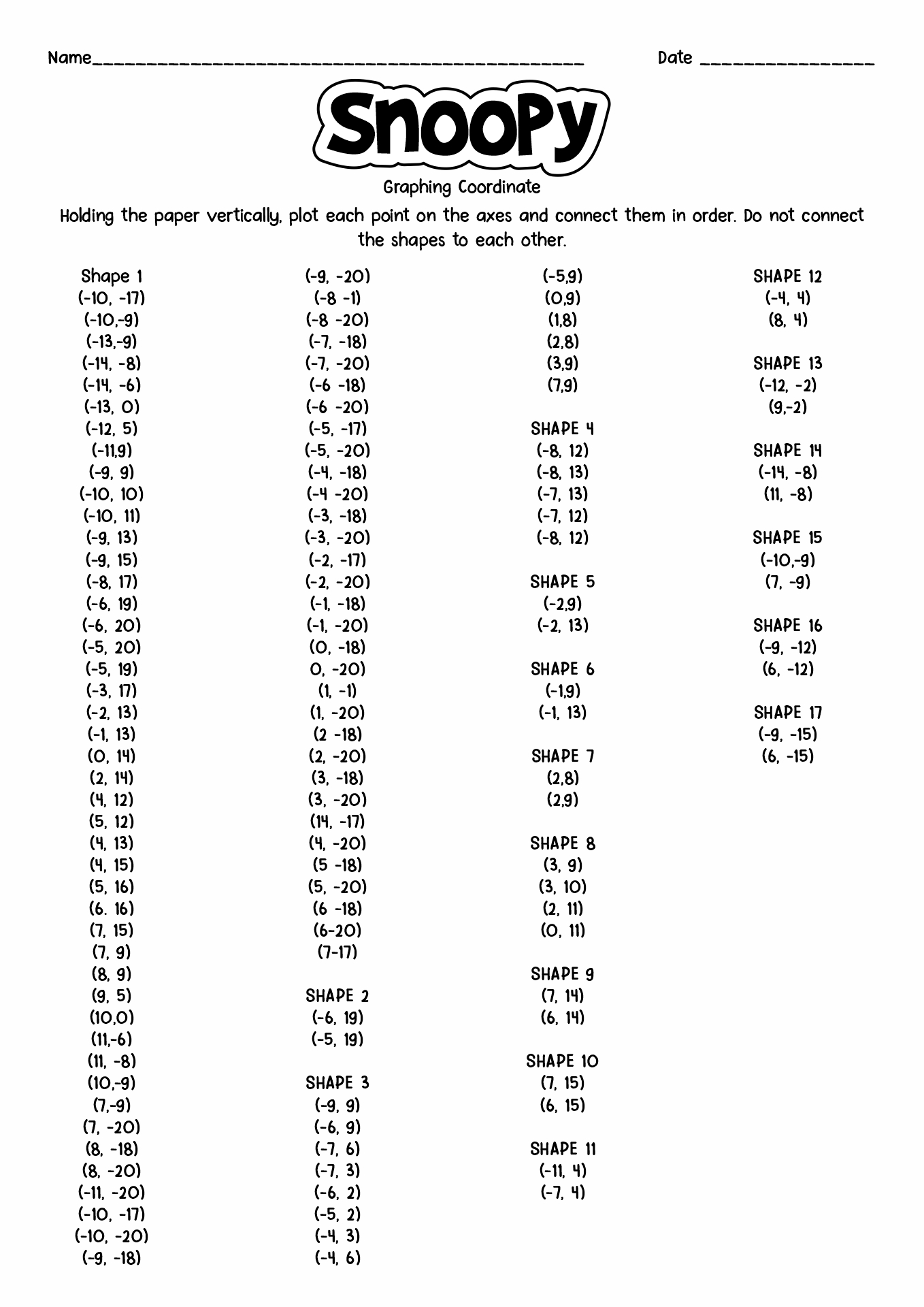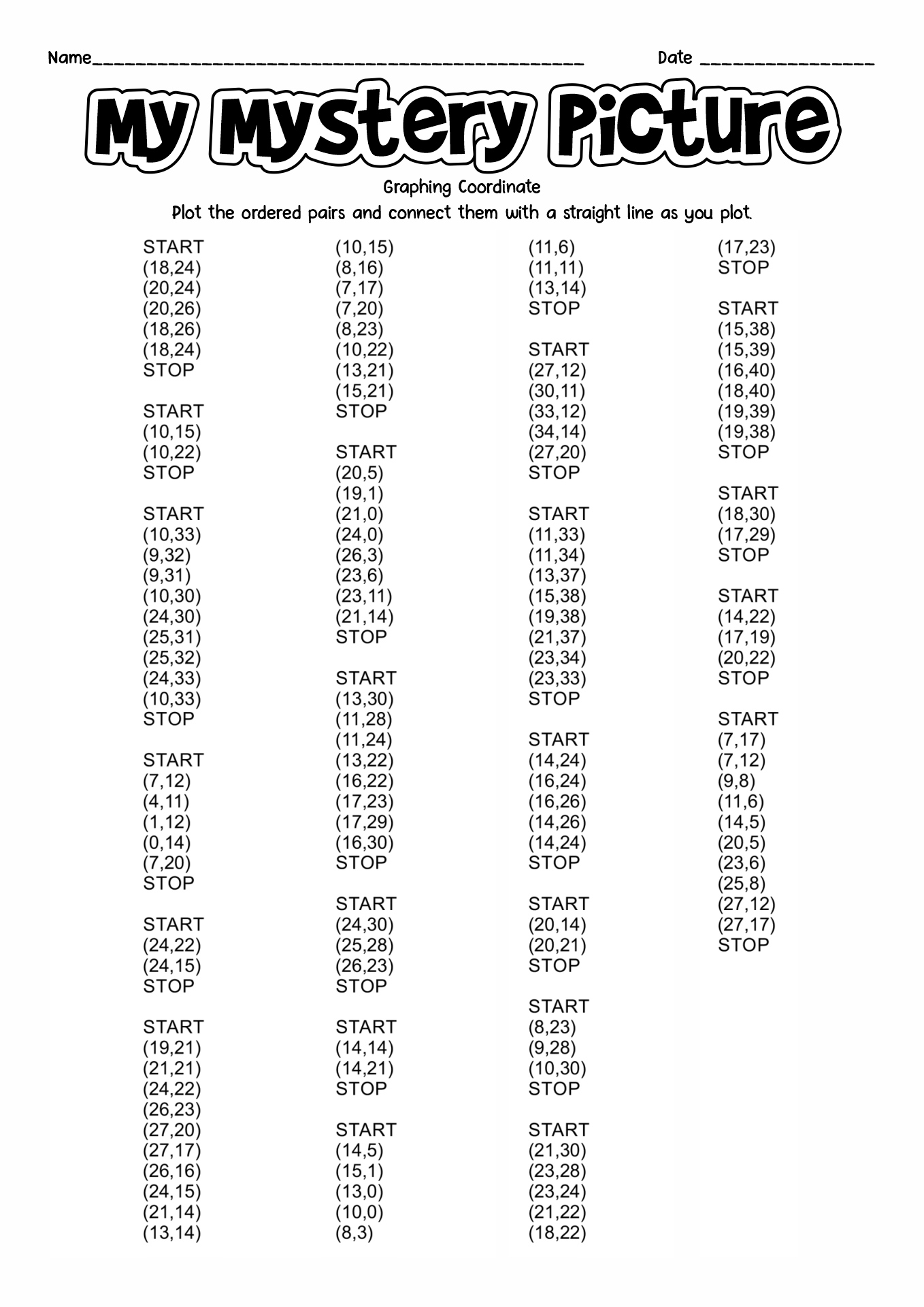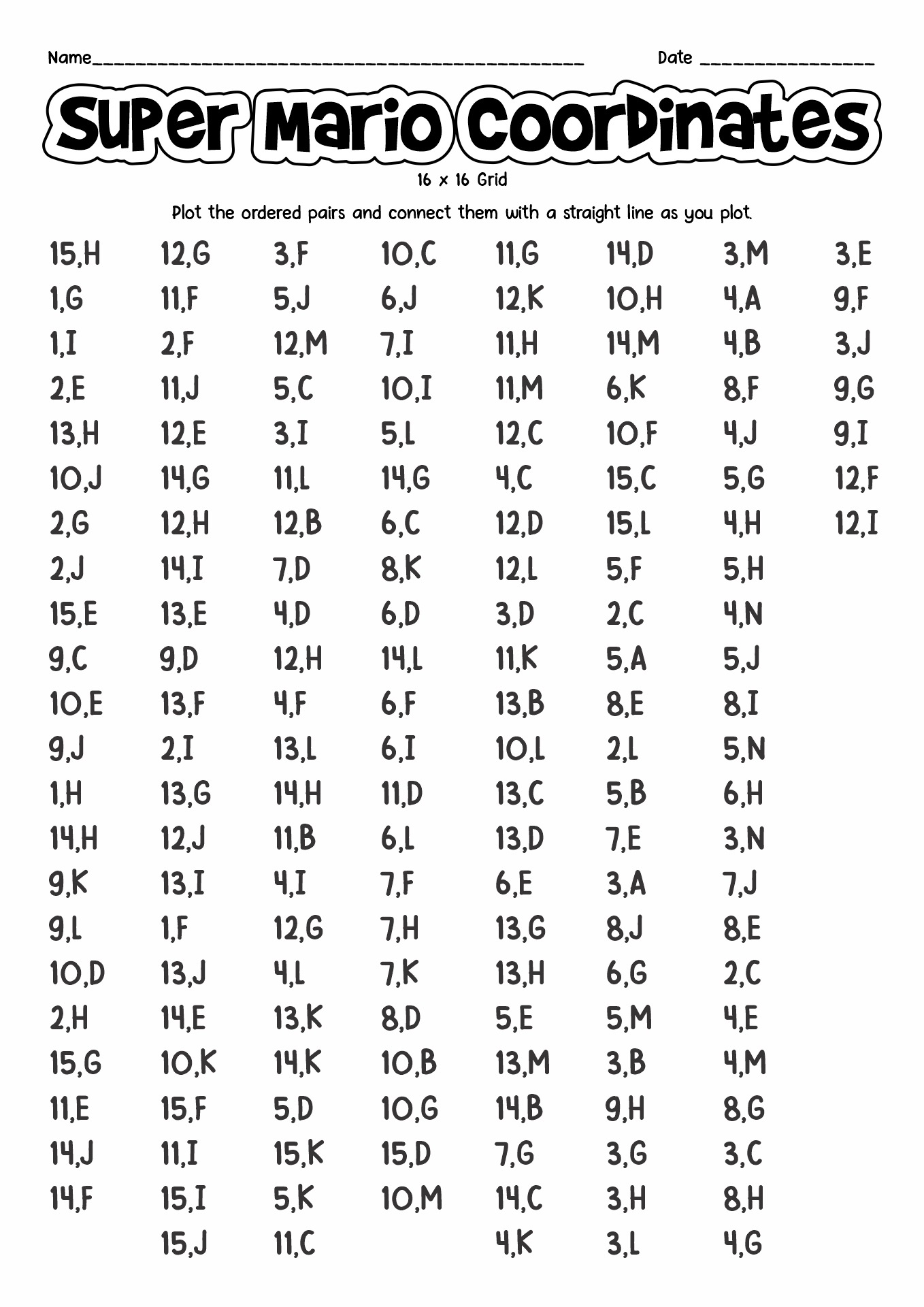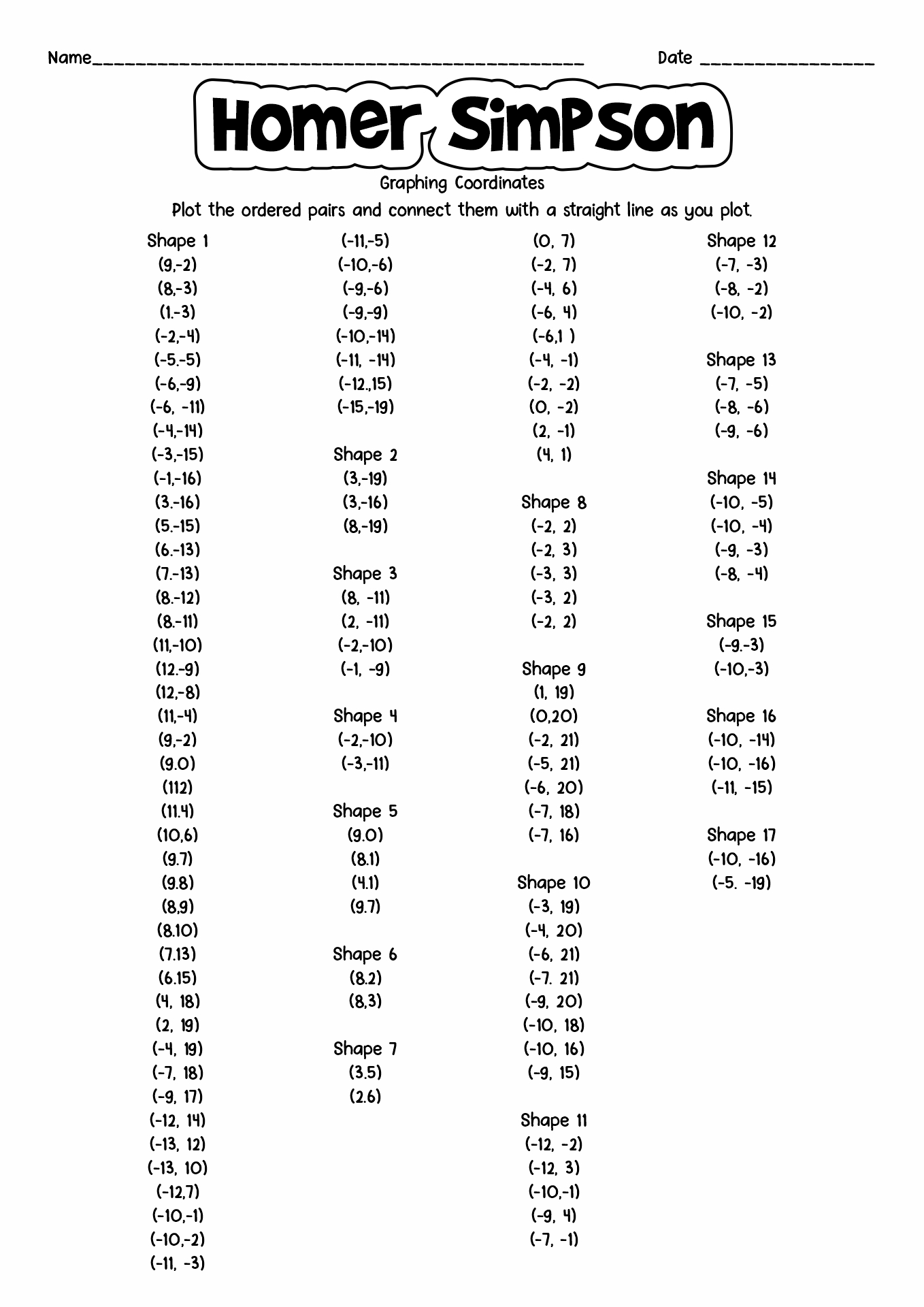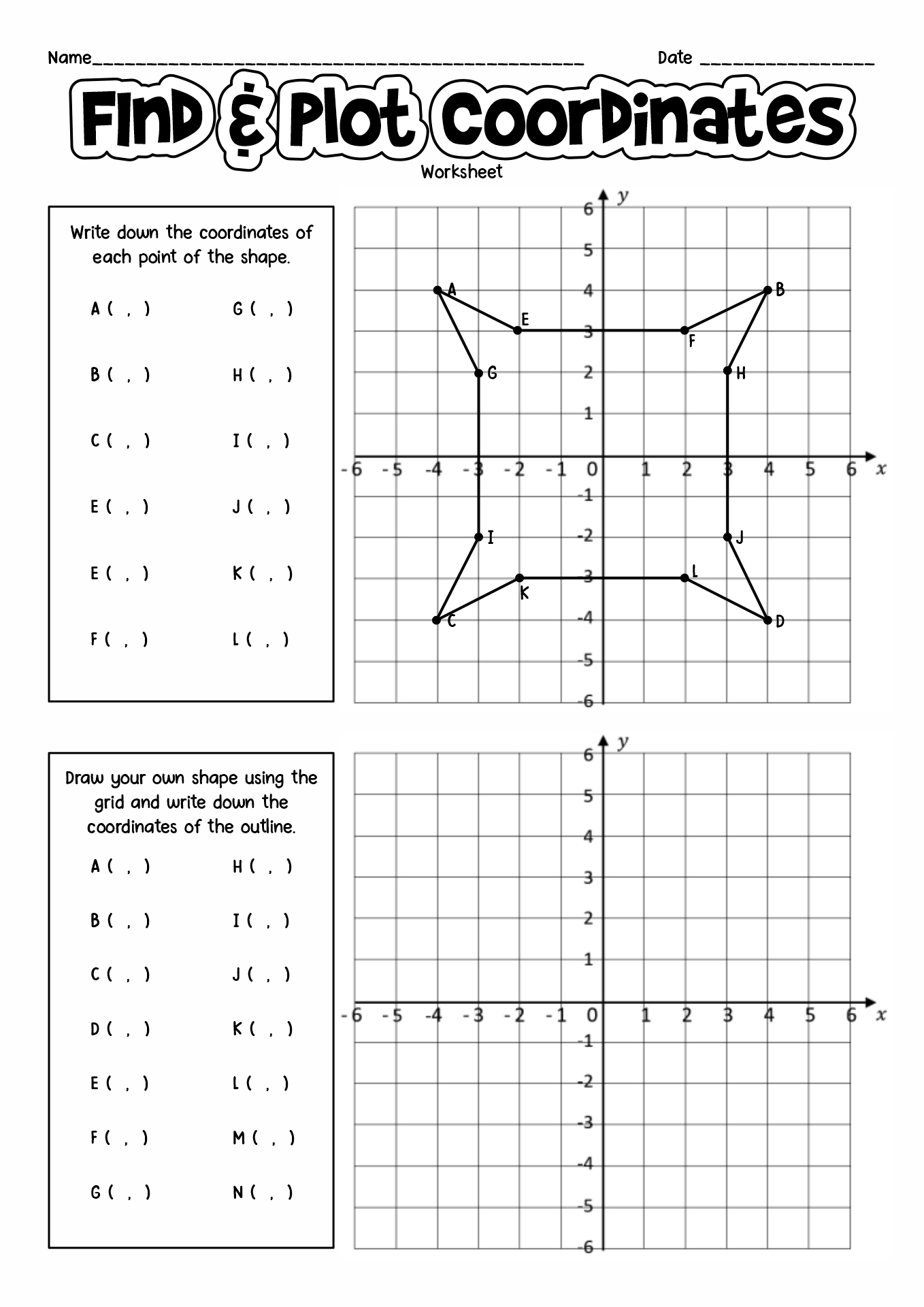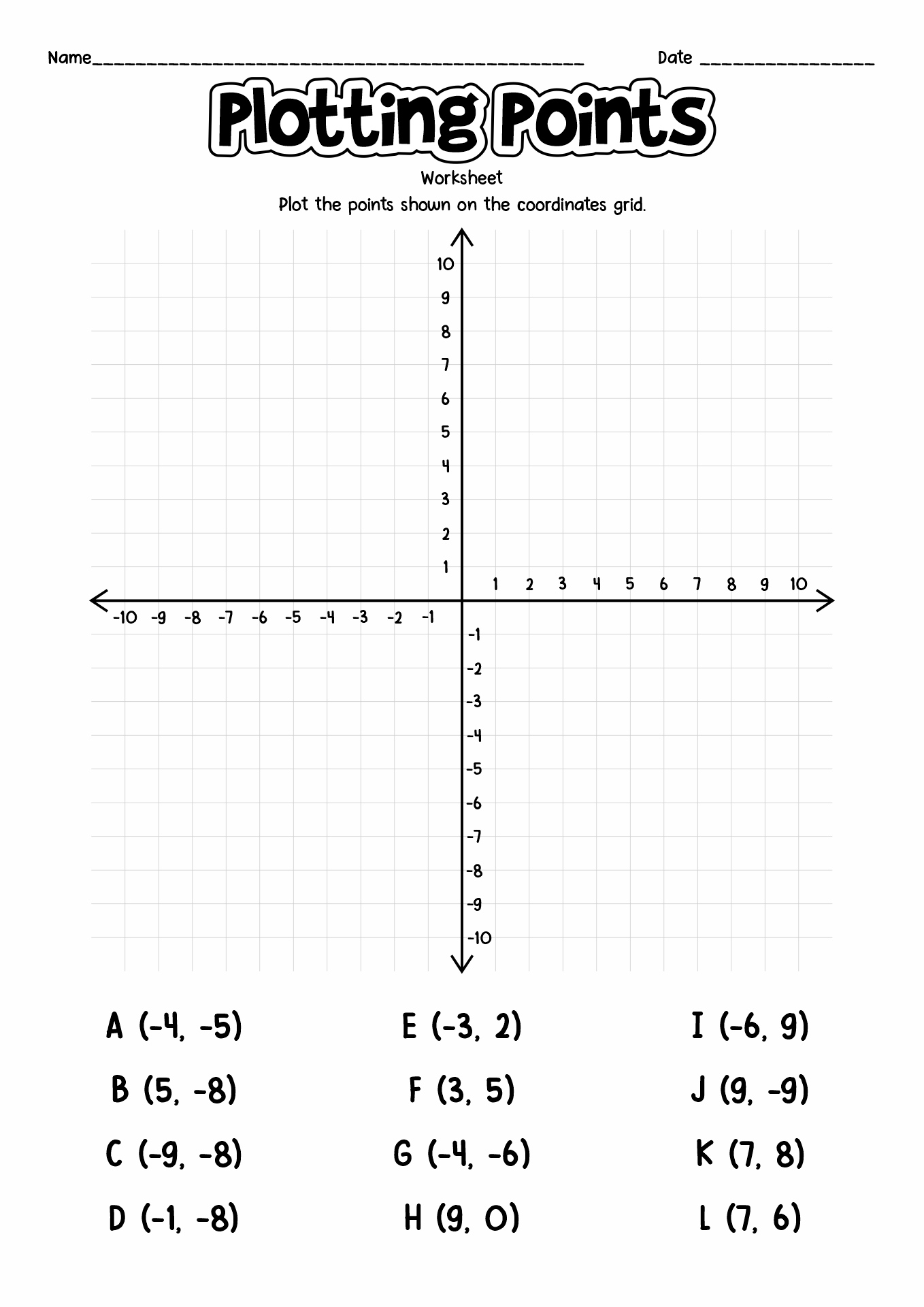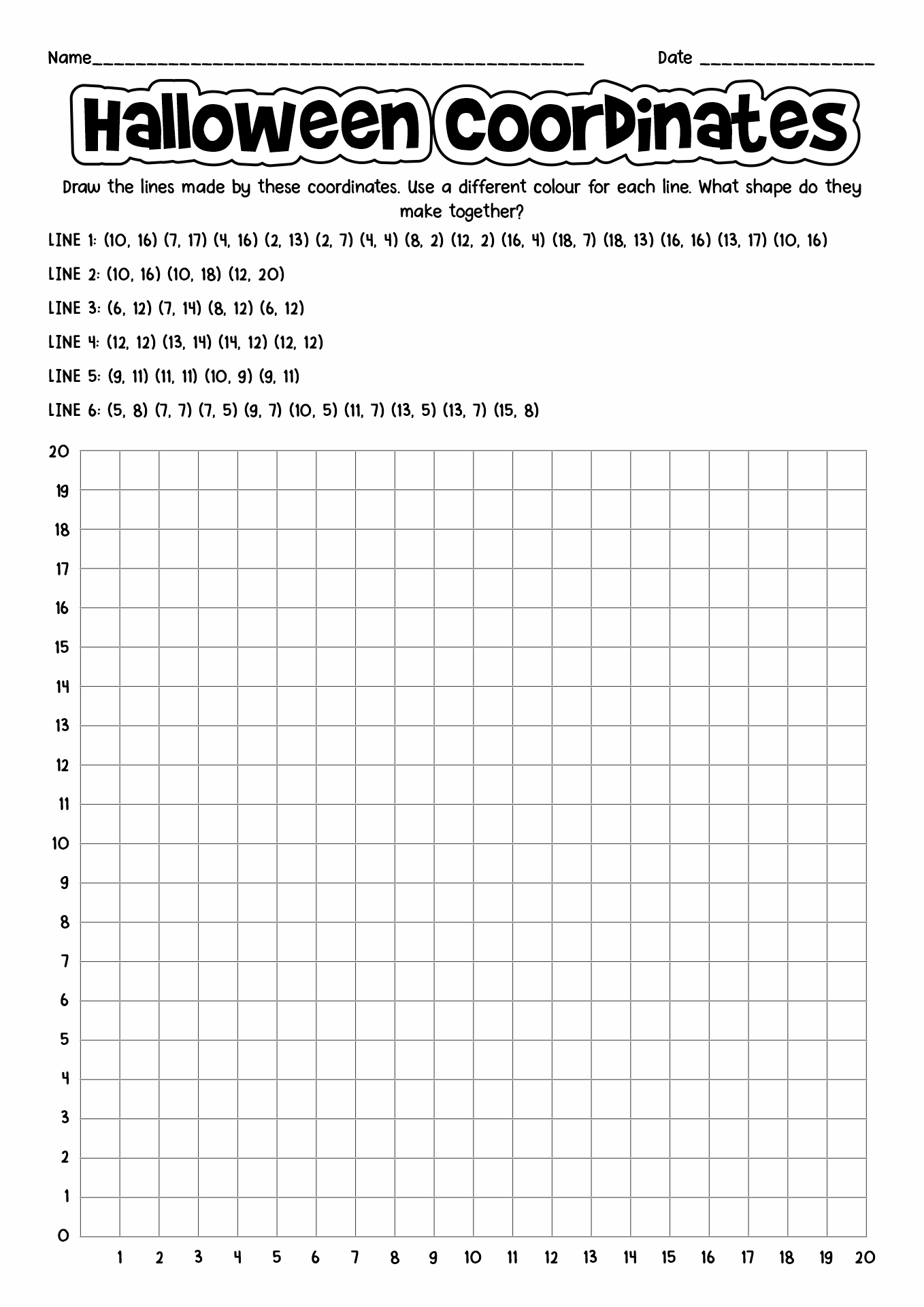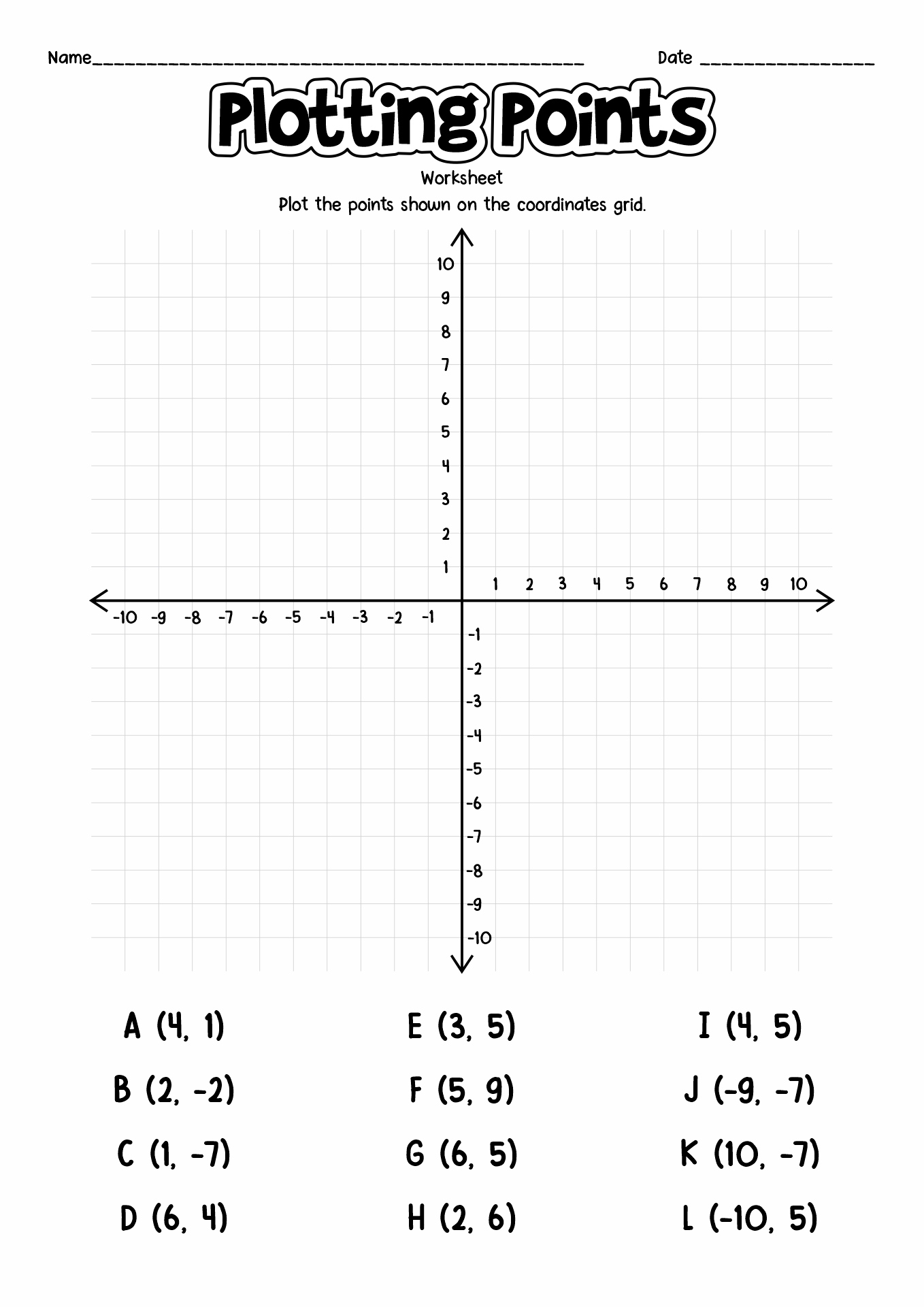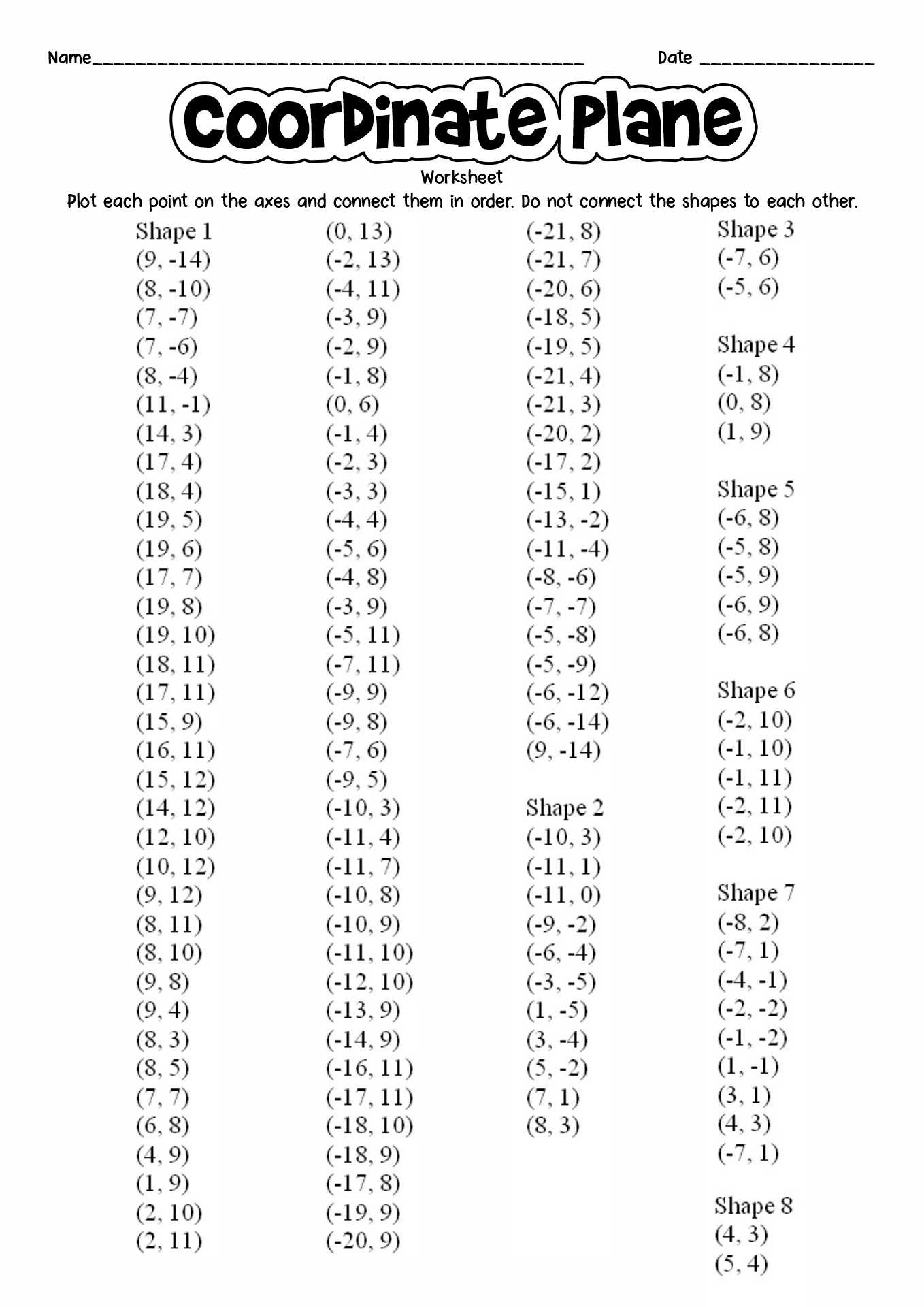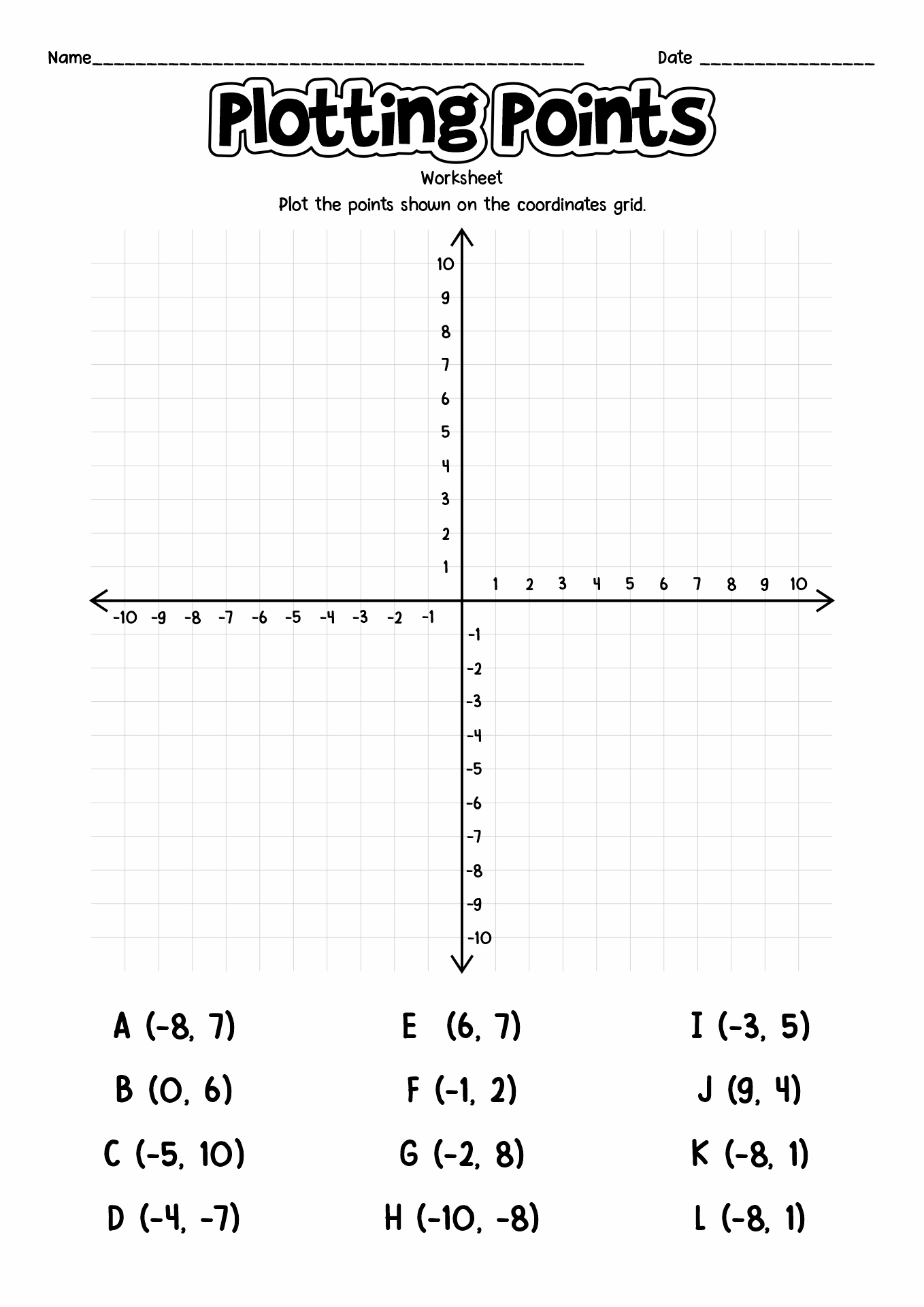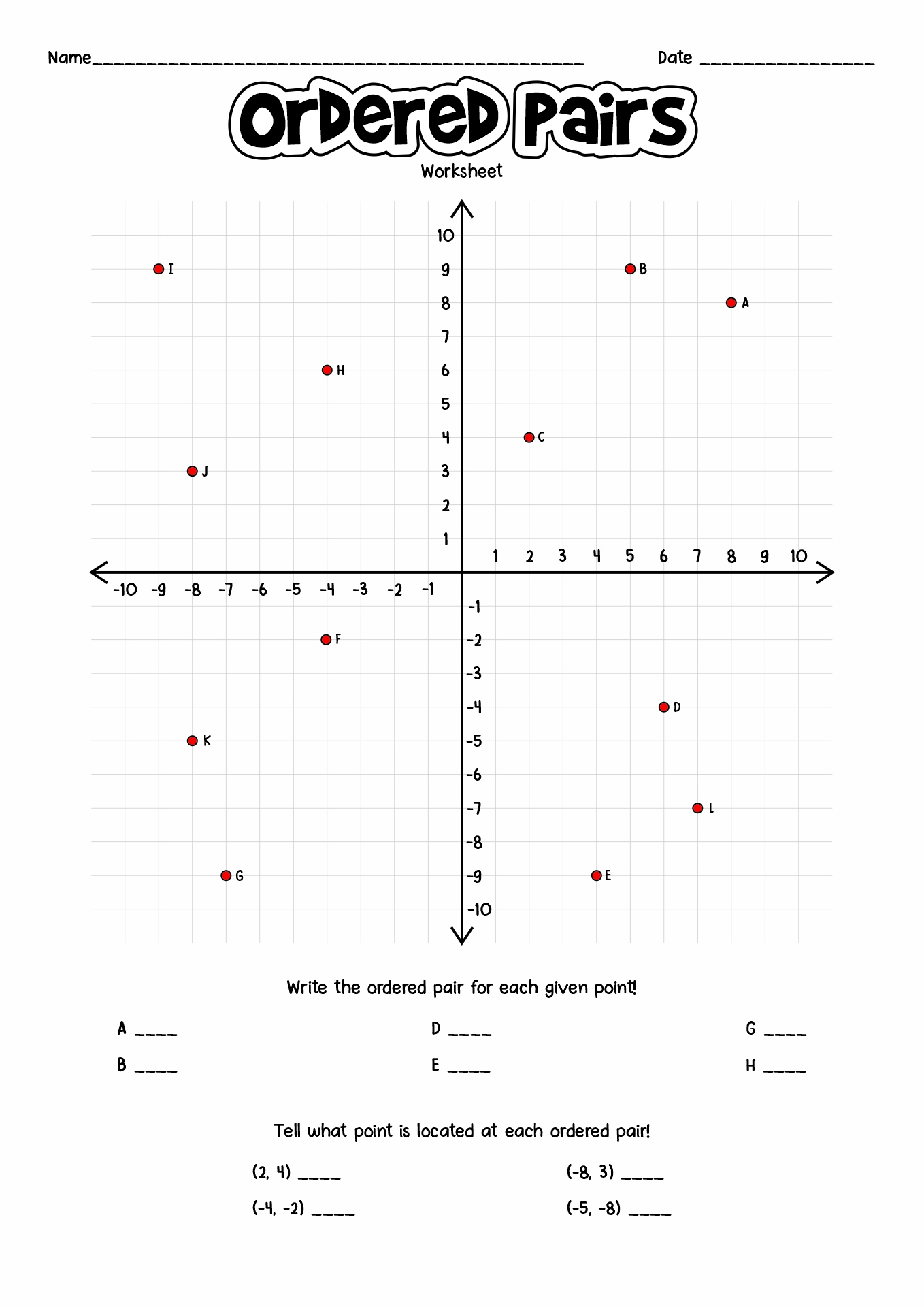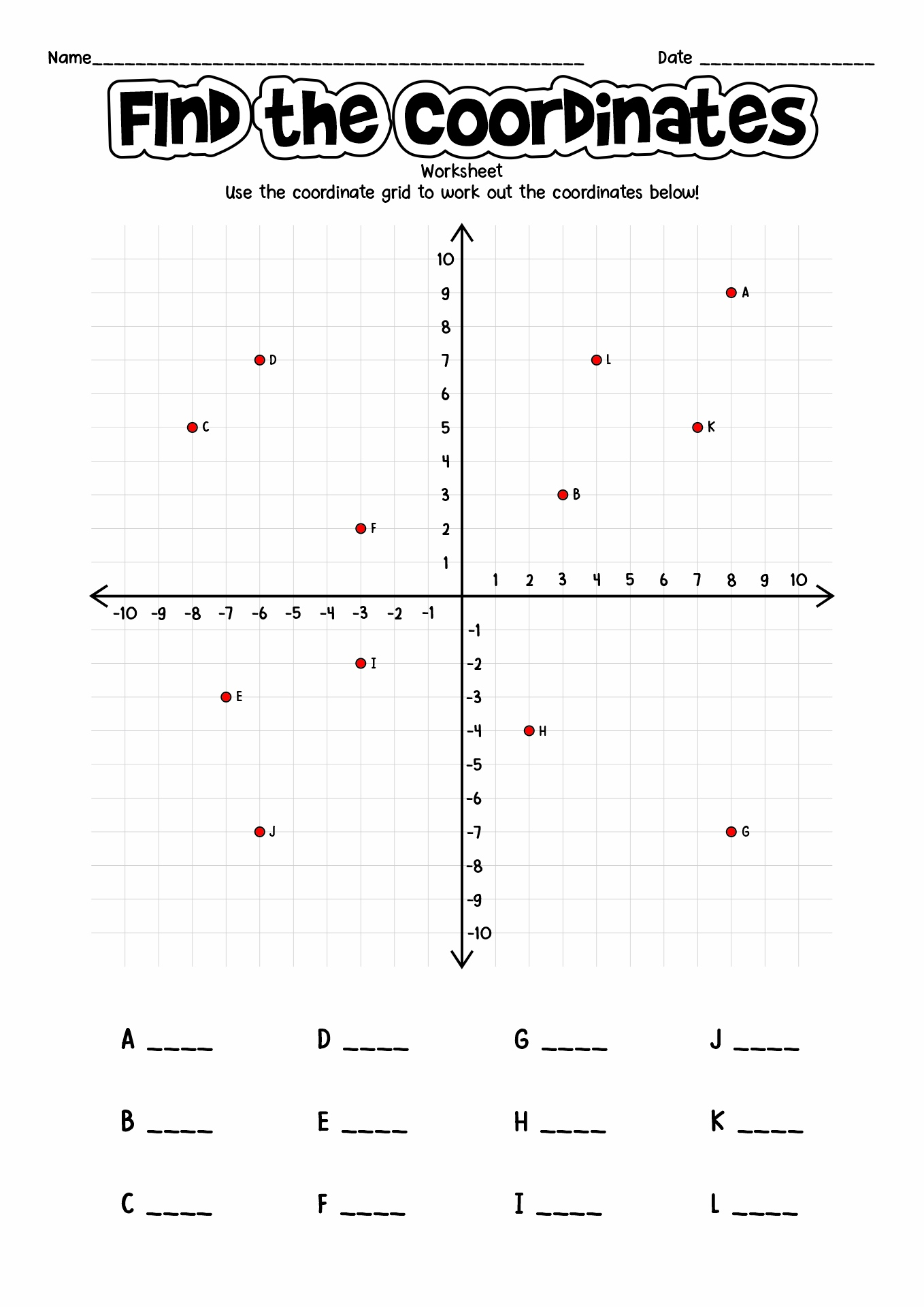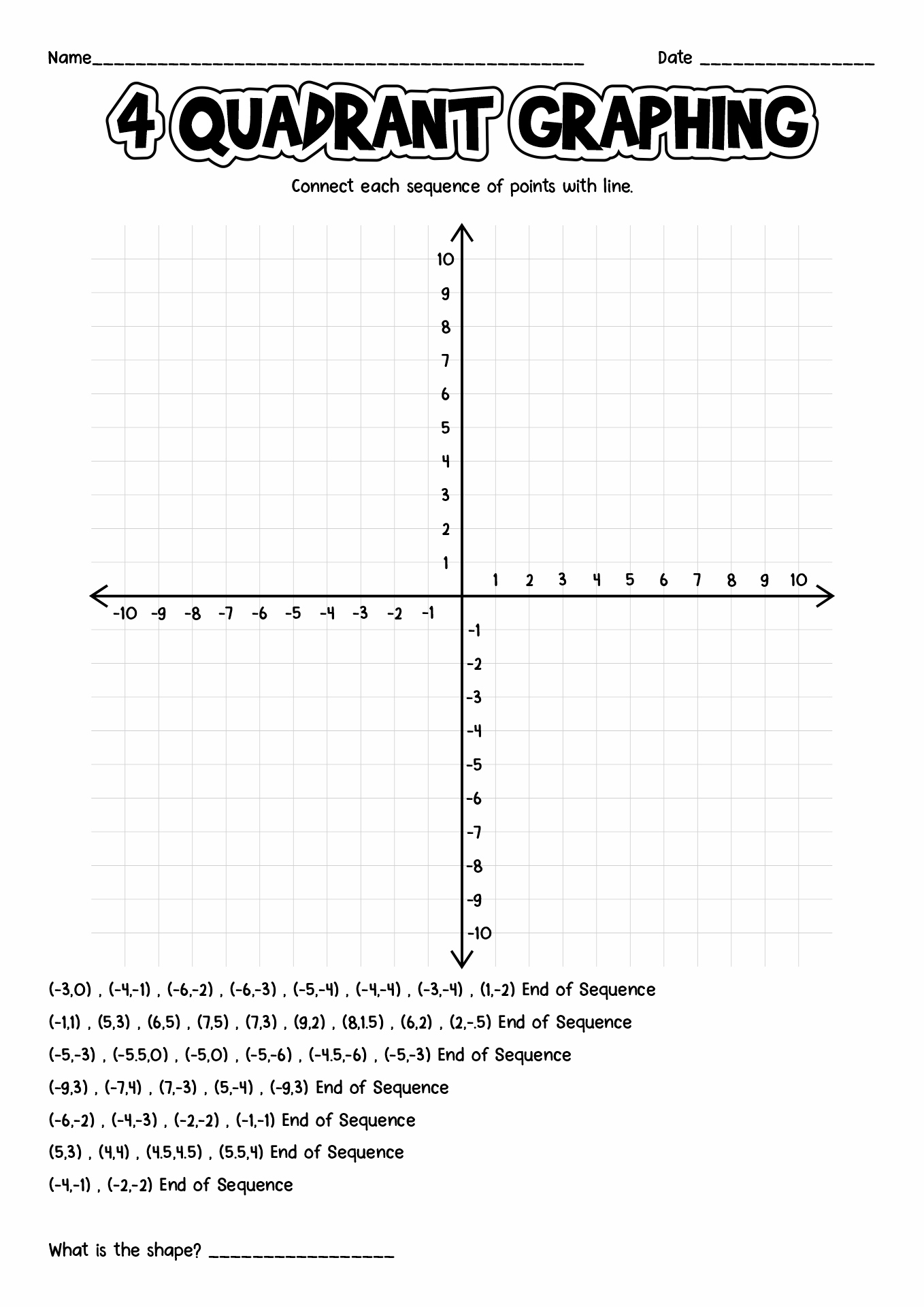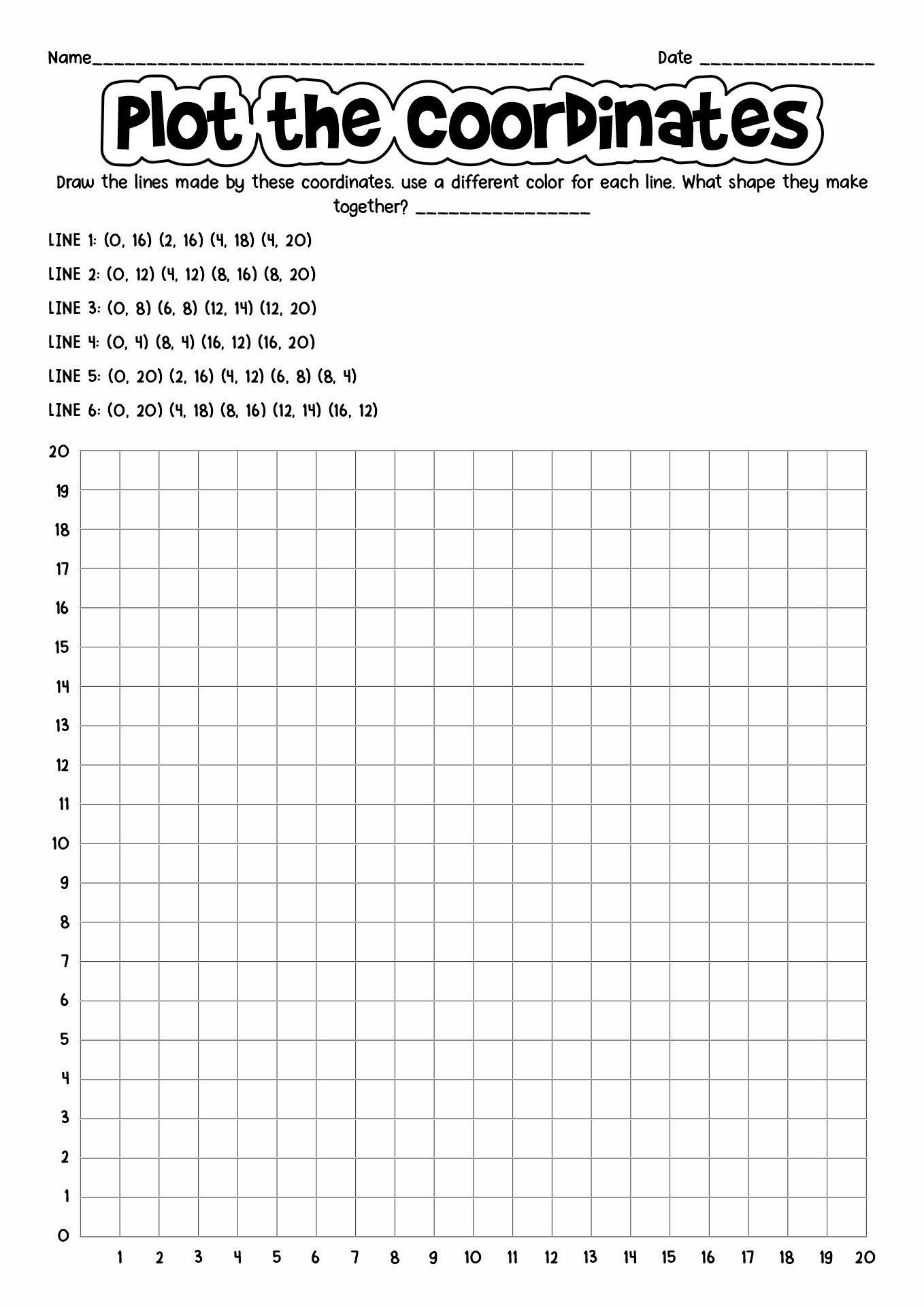### What is a Coordinate Plane?

Coordinate Plane or also known as a rectangular coordinate plane grid is a mathematical term in a form of a two-dimension surface formed by two number lines. The first line is a horizontal line called the x-axis, and the second line is a vertical line called the y-axis. These two lines crossed at the 0,0 point, called the origin. A coordinate plane can be used as a tool for graphing points, lines, and other objects. Not only as a theory on paper, coordinate planes also have many purposes in real life. Evangelista mentioned in her paper about coordinate plane usage in real life. Locating air transport, map projecting, GPS (Global Positioning System), and latitude and longitude function.

### How Do You Find the Coordinate in Coordinate Plane?

Plotting coordinates in a coordinate plane could be a tricky thing to learn and teach. here are some key points to understand how to plot coordinates by BBC UK.

• Coordinate plane is drawn on a pair of axes. The horizontal line is called the x-axis, and the vertical line is called the y-axis. Both of the lines crossed a path in the middle called the origin.

• Axes are labeled with numbers that are placed along the gridlines. Remember, the scales on the axes do not need to be the same.

• Coordinates are used to fix a position on a graph. A point is plotted using coordinates in the form (x,y).

• The x value gives the horizontal movement from the origin along the x-axis and the y value gives the vertical movement from the origin along the y-axis.

### What is the Right Time to Teach My Children About Coordinate Plane?

Universally, the coordinate plane is taught in Year 4 of elementary school. Usually, students are asked to describe positions on a 2D grid as coordinates in the first quadrant and plot specified points, and draw sides to complete a given polygon. When the students are in Year 6, the children will learn how to describe the positions of the full coordinate grid and start to draw and translate simple shapes on the coordinate plane, then reflect them on the axes. Teachers or parents could make this seemingly tough topic to learn, to be more enjoyable with Coordinate Plane Worksheet Spongebob!

### How to Teach Coordinate Plane to Young Learners?

Learning coordinate planes can be a boring activity for your students, so to make it more fun, teachers, and parents need to step up their games. You can start with some engaging graphing activities like Connect 4, Battleship, and Fence Graphing Activity. Other simpler activities that can be used to study coordinate plane are, identifying the given location through a coordinate plane, plotting locations on a coordinate plane, following and writing directions on a coordinate plane, measuring distances, and map scaling. Teachers and parents can download our Coordinate Plane Worksheets which are full of many cute and suitable illustrations for your children or students.

### What is Cartesian Coordinates System?

Michael DeMers explained the definition of cartesian coordinates as planar coordinate systems that define the location of any given point by a unique set of x and y coordinate pairs. The simpler explanation will be a two-line graph on which you plot ordered pairs. Cartesian coordinates are crucial tools for many applied practices, especially the one that deals with geometry, astronomy, physics, and so on. These coordinate systems let us to describes things like algebra in visual form, and help us to understand the concepts of algebra a lot easier.

### What is 4 Quadrant Graphing?

In the cartesian plane, the two intersecting lines create four distinct graph quadrants, this is what we called as a 4 quadrants graph. Shortly, the quadrant is the four sections that are divided by the coordinate axes. Each quadrant usually carries a mix of positive and negative values for x and y. Here are the graph quadrants and their values:

1. The first quadrant located in the upper right is called Quadrant I. The x-axis and y-axis have positive values in this quadrant.

2. The second quadrant located in the upper left is called Quadrant II. In this quadrant, the x-axis has negative values, meanwhile, the y-axis has positive values.

3. The third quadrant is located in the bottom left and is called Quadrant III. In this quadrant, both the x-axis and the y-axis have negative values on them.

4. The fourth is located in the bottom right and is called quadrant IV. The x-axis has positive values, and the y-axis has negative values in this quadrant.

### How to Make Learning Math Fun for Children?

It is no secret that learning math is scary and intimidating for some children. To overcome this dilemma, teachers and parent shall step up their techniques in teaching children. Using our Coordinate Plane Worksheets is one of the suggested approaches. The colorful worksheets completed by many cute children-favorite characters such as Spongebob, Olaf, Snoopy, Goofy, Superman, and Super Mario will make the kids motivated to learn.

The information, names, images and video detail mentioned are the property of their respective owners & source.

### Popular Categories

Have something to tell us about the gallery?

Submit